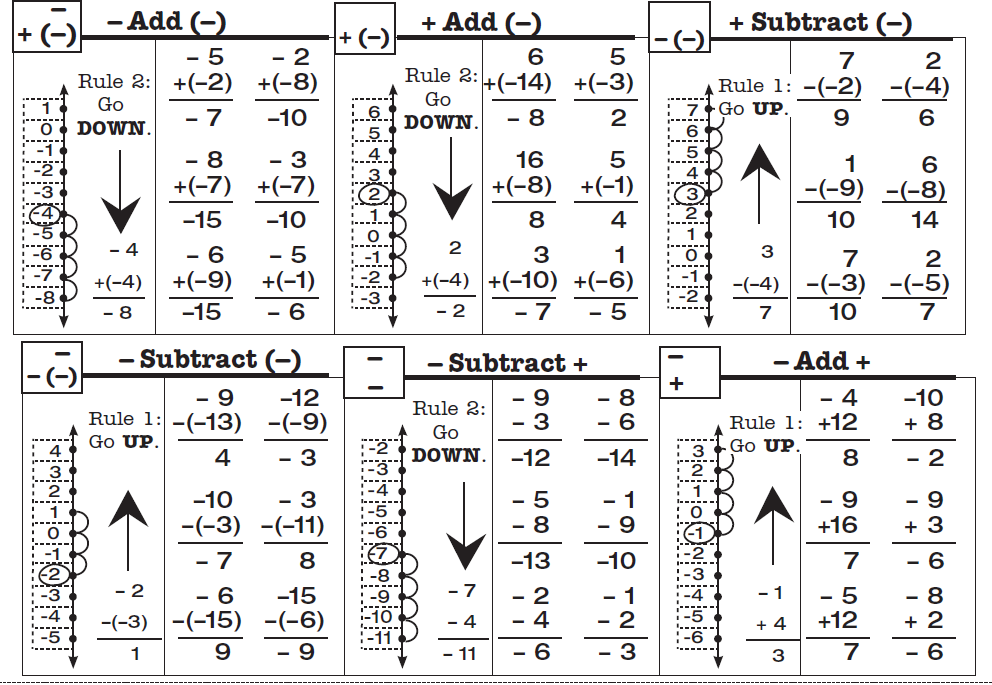## Mixed Integers combines Adding Integers and Subtracting Integers

If this mixed set goes too fast or is confusing for your students, an alternative is to start students with the two Learning Tracks that teach addition and subtraction separately.  The four addition processes are taught separately in the Learning to Add Integers Learning Track.   The four subtraction processes are taught separately in the Learning to Subtract Integers Learning Track.  If students have done those two Learning Tracks first, this will be a review and will be easier.

## Mixed Integers teaches eight processes and two rules.

Mixed Integers displays problems on a vertical number line and then teaches students two rules about how to solve problems that add or subtract positive and negative numbers.
Rule 1:  When you add a positive number OR subtract a negative number, go UP.
Rule 2:  When you subtract a positive number OR add a negative number, go DOWN.

Students learn how these rules play out when starting with a positive number and a negative number, gradually learning these two variations of all four types of problems.  They learn to solve a problem type using the number line and then to recognize the pattern of each problem type by working several examples of each type.  This practice gives them a chance to build fluency with each problem type as they work with their partner on the top half of the page.  You will probably not be surprised that there is a one-minute test on each set.  The goals are slightly different than before.  Students are to be 100% accurate and to meet or beat their goal from the special writing speed test for mixed integers.

## Video lessons teach the 8 processes.

There are 8 types of mixed integers problems (see below), between positive and negative numbers and the two operations. 8 online lessons teach students how each type of problem is solved and why it is correct.  (1) Mixed Integers Set A1 Positive add a positive

(2) Mixed Integers Set A2 Positive subtract a positive

(3) Mixed Integers Set D Negative add a positive

(4) Mixed Integers Set G Negative subtract a positive

(5) Mixed Integers Set J Negative subtract a negative

(6) Mixed Integers Set M Positive subtract a negative

(7) Mixed Integers Set P Positive add a negative

(8) Mixed Integers Set S Negative add a negative